The MODEL Procedure

## Example 18.19 EMM Estimation of a Stochastic Volatility Model

The efficient method of moments (EMM), introduced by Bansal et al. (1993 and 1995) and Gallant and Tauchen (2001), can be considered a variant of SMM. The idea is to match the efficiency of the maximum likelihood (ML) estimation with the flexibility of the SMM procedure. ML itself can be interpreted as a method of moments procedure, where the score vector, the vector of derivatives of the log-likelihood function with respect to the parameters, provides the exactly identifying moment conditions. EMM employs an auxiliary (or pseudo) model that closely matches the true model. The score vector of the auxiliary model provides the moment conditions in the SMM step.

This example uses the SMM feature of PROC MODEL to estimate the simple stochastic volatility (SV) model of Example 18.17 with the EMM method.

Suppose that your data are the time series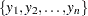, and the model that you want to estimate, or the structural model, is characterized by the vector of parameters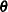. For the SV model,is given by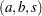.

The first step of the EMM method is to fit the data with an auxiliary model (or score generator) that has transition density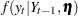, parametrized by the pseudo parameter
, where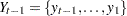. The auxiliary model must approximate the true data-generating process as closely as possible and be such that ML estimation is feasible.

The only identification requirement is that the dimension of the pseudo parameterbe greater than or equal to that of the structural parameter.

Andersen, Chung, and Sorensen (1999) showed that the GARCH(1,1) is an appropriate auxiliary model that leads to a good performance of the EMM estimator for the SV model.

The analytical expression for the GARCH(1,1) model with mean zero is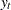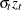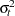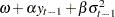The pseudo parameter vectoris given by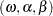.

One advantage of such a class of models is that the conditional density ofis Gaussian—that is,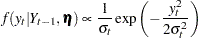Therefore the score vector can easily be computed analytically.

The AUTOREG procedure provides the ML estimates,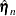. The output is stored in the garchout data set, while the estimates are stored in the garchest data set.

```title1 'Efficient Method of Moments for Stochastic Volatility Model';

/* estimate GARCH(1,1) model */
proc autoreg data=svdata(keep=y)
outest=garchest
noprint covout;
model y =  / noint garch=(q=1,p=1);
output out=garchout cev=gsigmasq r=resid;
run;
```

If the pseudo model is close enough to the structural model, in a suitable sense, Gallant and Long (1997) showed that a consistent estimator of the asymptotic covariance matrix of the sample pseudo-score vector can be obtained from the formula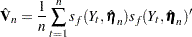where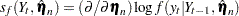denotes the score function of the auxiliary model computed at the ML estimates.

The ML estimates of the GARCH(1,1) model are used in the following SAS statements to compute the variance-covariance matrix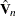.

```/* compute the V matrix */
data vvalues;
set garchout(keep=y gsigmasq resid);

/* compute scores of GARCH model */
score_1 = (-1 + y**2/gsigmasq)/ gsigmasq;
score_2 = (-1 + y**2/gsigmasq)*lag(gsigmasq) / gsigmasq;
score_3 = (-1 + y**2/gsigmasq)*lag(y**2) / gsigmasq;

array score{*} score_1-score_3;
array v_t{*} v_t_1-v_t_6;
array v{*} v_1-v_6;

/* compute external product of score vector */
do i=1 to 3;
do j=i to 3;
v_t{j*(j-1)/2 + i} = score{i}*score{j};
end;
end;

/* average them over t */
do s=1 to 6;
v{s}+ v_t{s}/&nobs;
end;
run;
```

The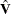matrix must be formatted to be used with the VDATA= option of the MODEL procedure. See the section VDATA= Input data set for more information about the VDATA= data set.

```/* Create a VDATA dataset acceptable to PROC MODEL */

/* Transpose the last obs in the dataset */
proc transpose data=vvalues(firstobs=&nobs keep=v_1-v_6)
out=tempv;
run;

/* Add eq and inst labels */
data vhat;
set tempv(drop=_name_);
value = col1;
drop col1;
input _type_ \$ eq_row \$ eq_col \$ inst_row \$ inst_col \$; *\$;
datalines;
gmm m1 m1 1 1  /* intcpt is the only inst we use */
gmm m1 m2 1 1
gmm m2 m2 1 1
gmm m1 m3 1 1
gmm m2 m3 1 1
gmm m3 m3 1 1
;
```

The last step of the EMM procedure is to estimateby using SMM, where the moment conditions are given by the scores of the auxiliary model.

Given a fixed value of the parameter vectorand an arbitrarily large T, one can simulate a series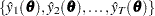from the structural model. The EMM estimator is the value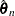that minimizes the quantity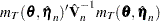where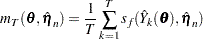is the sample moment condition evaluated at the fixed estimated pseudo parameter. Note that the target function depends on the parameteronly through the simulated series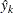.

The following statements generate a data set that contains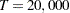replicates of the estimated pseudo parameterand that is then input to the MODEL procedure. The EMM estimates are found by using the SMM option of the FIT statement. Thematrix computed above serves as weighting matrix by using the VDATA= option, and the scores of the GARCH(1,1) auxiliary model evaluated at the ML estimates are the moment conditions in the GMM step.

Since the number of structural parameters to estimate (3) is equal to the number of moment equations (3) times the number of instruments (1), the model is exactly identified and the objective function has value zero at the minimum.

For simplicity, the starting values are set to the true values of the parameters.

```/* USE SMM TO FIND EMM ESTIMATES */

/* Generate dataset of length T */
data emm;
set garchest(obs=1 keep = _ah_0 _ah_1 _gh_1 _mse_);
do i=1 to 20000;
output;
end;
drop i;
run;

title2 'EMM estimates';
/* Find the EMM estimates */
proc model data=emm maxiter=1000;
parms a -0.736 b 0.9 s 0.363;
instrument _exog_ / intonly;

/* Describe the structural model */
u = rannor( 8801 );
z = rannor( 9701 );
lsigmasq = xlag(sigmasq,exp(a));
lnsigmasq = a + b * log(lsigmasq) + s * u;
sigmasq = exp( lnsigmasq );
ysim = sqrt(sigmasq) * z;

/* Volatility of the GARCH model */
gsigmasq = _ah_0 + _gh_1*xlag(gsigmasq, _mse_)
+ _ah_1*xlag(ysim**2, _mse_);

/* Use scores of the GARCH model as moment conditions */
eq.m1 = (-1 + ysim**2/gsigmasq)/ gsigmasq;
eq.m2 = (-1 + ysim**2/gsigmasq)*xlag(gsigmasq, _mse_) / gsigmasq;
eq.m3 = (-1 + ysim**2/gsigmasq)*xlag(ysim**2, _mse_) / gsigmasq;

/* Fit scores using SMM and estimated Vhat */
fit m1 m2 m3 / gmm npreobs=10 ndraw=1 /* smm options */
vdata=vhat /* use estimated Vhat */
kernel=(bart,0,) /* turn smoothing off */;
bounds s > 0, 1>b>0;
run;
```

The output of the MODEL procedure is shown in Output 18.19.1.

Output 18.19.1 PROC MODEL Output
 Efficient Method of Moments for Stochastic Volatility Model EMM estimates

The MODEL Procedure

Model Summary
Parameters 3
Equations 3
Number of Statements 11

Parameters(Value) a(-0.736) b(0.9) s(0.363) m1 m2 m3

The 3 Equations to Estimate
m1 = F(a, b, s)
m2 = F(a, b, s)
m3 = F(a, b, s)
Instruments 1

Nonlinear GMM Parameter Estimates
Parameter Estimate Approx Std Err t Value Approx
Pr > |t|
a -0.56165 0.0160 -35.11 <.0001
b 0.921532 0.00217 425.26 <.0001
s 0.344669 0.00674 51.11 <.0001Previous Page | Next Page | Top of Page